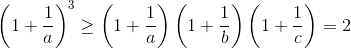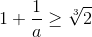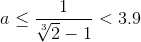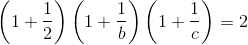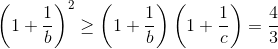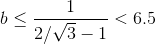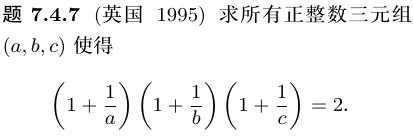1. 首先思考两变量的情况，即：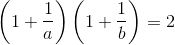2. 因为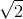是无理数， a = b 是不可能的。
3. 心算化简：
• (a + 1)(b + 1) = 2ab
• a + b + 1 = ab
• a + 1= (a - 1)b
• b = (a + 1) / (a - 1)
4. 很显然，(a, b) = (2, 3) 是一组解。
5. 既然两变量的情况有解，三变量的原题也可能有解。
6. 先固定 c = 2，得到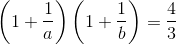7. 再试试 c = 3，得到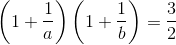8. 继续试 c = 4，得到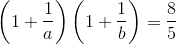9. 嗯，还是 c = 3 的情况最简单。
10. 类似地心算化简：
• 2(a + 1)(b + 1) = 3ab
• 2a + 2b + 2 = ab
• 2a + 2= (a - 2)b
• b = 2(a + 1) / (a - 2)
11. 显然，原方程有以下解：
• (a,b,c) = (3,8,3)
• (a,b,c) = (4,5,3)
• (a,b,c) = (5,4,3)
• (a,b,c) = (8,3,3)

• (a,b,c) = (4,15,2)
• (a,b,c) = (5,9,2)
• (a,b,c) = (6,7,2)

``````#include <stdio.h>

int main(void)
{
const int n = 1000;
for (int a = 2; a <= n; a++)
for (int b = a; b <= n; b++)
for (int c = b; c <= n; c++)
if (2L*a*b*c == (a+1L)*(b+1)*(c+1))
printf("%d,%d,%d\n", a, b, c);
}
``````

2,4,15
2,5,9
2,6,7
3,3,8
3,4,5